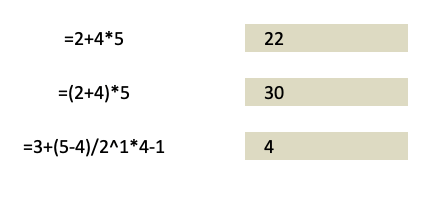When working with calculations with Excel Pivot Tables, you need to be aware of the Order of Operations so that you will not get confused about how it arrived at the final output.

Throughout the entire journey from basic to advanced mathematics, one certain rule has always stuck with us. This rule is commonly known as PEMDAS, namely – Parentheses, Exponents, Multiplication, Division, Addition, and Subtraction.

This rule tells us the correct sequence, or order of steps while evaluating a mathematical expression.

A simple expression like 4+5 with only one operator is simple to evaluate, but a more complex expression such as 4*5+6 requires us to follow a certain fixed rule, where we evaluate the expression following a set sequence. This sequence is provided by PEMDAS. In this example, according to PEMDAS, the order is first multiplication, then subtraction.

=4*5+6

=20+6

=26

Similarly, Excel Pivot Table also follows such an order of operations for evaluating expressions. It is somewhat similar to what we have learned in our PEMDAS rule, but with a few adjustments to accommodate for the variances and formula syntax while working on a spreadsheet.

Keeping in view a few adjustments, the rule or “order of operations” that excel follows is:

• Brackets
• Reference operators
• Exponents
• Percentages
• Multiplication and Division
• Addition and Subtraction
• Concatenation (&)
• Comparisons (> < = <>)

Excel evaluates any expression that is within parentheses first. This is generally true for any expressions, as parentheses override the order of operations and are evaluated first. Inside the parentheses, the normal order of operations is followed.

Example: =(5*6)+1

= 30+1

=31

Secondly, Excel evaluates any reference operators. Reference operators are any expressions that reference a certain cell (like A1) or a certain range (like A1:B10). It replaces the cell references with the referred cell’s values and then carries on with the remaining operations.

Example: = B12/2 + 10

= 20/2 +10 (where cell B12 contains value 20)

= 10+10

= 20

Next, Excel evaluates exponents.

Example: =2^2

=4

It is followed by evaluating percentage conversions.

Example: = 20% +10

= 0.2+10

= 10.2

Next in order are the general mathematical operators that are evaluated in the order of Multiplication and Division, and then Addition and Subtraction. If there is an expression that contains multiple operators of the same priority like multiplication and division together, Excel will evaluate these operators from left to right.

Example: = 4*5-6

= 20-6

= 14

In the end, Excel evaluates uncommon operators like concatenation and logical operators. Excel first evaluates any concatenation present in the expression.

Example: = “Total: “&10+25

=Total: 35

Lastly, it works on any logical operators.

Example: = 3>5

=FALSE

Now that you know the order of operations that Excel follows, let’s look at different examples to understand it even better!

Don’t forget to download the Exercise Workbook below and follow along with us.

These are the 3 examples that we want to assess and evaluate step by step.Example #1

Let us check the first example: =2+4*5

There are 2 operations here so based on the table above, the order of operations will be: Multiplication then addition

• =2+4*5
• =2+20
• =22

Example #2

Let us check the second example: =(2+4)*5

There are 3 operations here so based on the table above, the order of operations will be: Brackets (i.e. addition) then multiplication.

• =(2+4)*5
• =6*5
• =30

Example #3

Let us check the third example: 3+(5-4)/2^1*4-1

• Firstly, Excel will evaluate the expression within the parentheses, reducing the expression to
= 3+1 / 2^1*4-1
• Next, Excel will calculate the exponential i.e. 2^1=2
= 3+1 / 2*4-1
• Excel will then calculate multiplications and division from left to right i.e. 1/2*4 i.e. 2
= 3+2-1
• Finally, we have our addition and subtraction, which is also done from left to right
= 4

So, by going through these examples we have understood the order of operations that Excel follows while evaluating any expression. Refer to this sequence whenever you are building an expression to get accurate results.